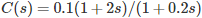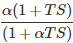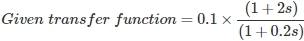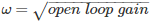# MCQs on Control Systems

##### Page 23 of 23. Go to page 1 2 3 4 5 6 7 8 9 10 11 12 13 14 15 16 17 18 19 20 21 22 23
01․ With regard to filtering property, the lead compensator is
band reject filter

Lead compensator characteristics: 1. For sinusoidal input, the phase of controller output leads by tan-1 (ωTd). 2. In terms of filtering property it acts as high pass filter. 3. It reduces rise time. 4. It increases bandwidth. 5. It increases stability of the system. 6. It reduces peak over shoot.

02․ A controller transfer function is given by. What is its nature and parameter?
lag compensator, β = 10
lag compensator, β = 2

The transfer function of phase lead compensator =Where, α < 1 Therefore for lead compensator zero is nearer to origin.By comparing two transfer functions, T = 2 and α = 0.1. Therefore, given transfer function is lead compensator.

03․ Which of the following is/are represents the lead compensator?
(s+2)/(s+1)
(s+2)/(s+5)
(s+5)/(s+6)
both 2 and 3

The transfer function of phase lead compensator = α(1 + TS)/(1 + αTS) Where α < 1 Therefore for lead compensator zero is nearer to origin.

04․ Which of the following is/are represents the lag compensator?
(s+2)/(s+1)
(s+2)/(s+5)
(s+5)/(s+6)
both 1 and 2

The transfer function of phase lag compensator = (1 + TS)/(1 + βTS) Where β > 1 Therefore for lag compensator pole is nearer to origin.

05․ The transfer function of a phase lead compensator is given by: G(s) = (1 + 3Ts)/(1 + Ts) where T>0. What is the maximum phase shift provided by such a compensator?
90°
45°
30°
60°

Maximum phase shift φm = sin-1((1 - α)/(1 + α) Given transfer function G(s) = (1 + 3Ts)/(1 + Ts) From the above transfer function α = 1/3 Maximum phase shift φm = sin-1((1-1/3)(1+1/3)) Maximum phase shift φm = 30°

06․ The following transfer function represents a phase-lead compensator?
(s+4)/(s+6)
(4s+2)/(6s+1)
(s+4)/(3s+6)
1/s

The transfer function of phase lead compensator = α(1 + TS)/(1 + αTS) Where α < 1 Therefore for lead compensator zero is nearer to origin.

07․ To achieve the optimum transient response, the indicating instruments are so designed as to
be undamped.
be critically damped.
provide damping which is slightly less than the critical value.
provide damping which is slightly more than the critical value.

To achieve the optimum transient response, the indicating instruments are provided with a damping which is slightly less than the critical value.

08․ The initial slope of Bode plot for a transfer function having no poles at the origin is

Since there are no poles at the origin, so it is a TYPE I system. So the initial slope is 0 dB/decade.

09․ The low frequency and high frequency asymptotes of Bode magnitude plot are respectively -60dB/decade and -40dB/decade. What is the type of the system?
TYPE 0
TYPE II
TYPE III
TYPE I

The low frequency of Bode magnitude plot is -60dB/decade. So there are three plose at the origin. So the system is TYPE III.

10․ At which frequency does the Bode magnitude plots for the functions K/S2 have gain crossover frequency
The gain crossover frequency for a TYPE II system is given by. Here open loop gain = K. So the gain crossover frequency is ω = √K rad/sec.